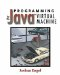# 6.3 Are All Constant References Correct?

After asking whether or not the file looks like a properly formatted class file, the verification algorithm knows where the constant pool is to be found and how many constants are in it. It also knows that all constant tags are valid. (If there was an invalid constant tag, it wouldn't know how many bytes that constant took up, and it would have rejected the file.) Now it can ask the question "Are the constants themselves correctly formed?" That is,

• Do Class and String constants have a reference to another constant that is a UTF8 constant?

• Do Fieldref, Methodref, and InterfaceMethodref constants have a class index that is a Class constant and a name-and-type index that is a NameAndType constant?

• Do NameAndType constants have a name index that points to a UTF8 and a type index that points to a UTF8?

• Does the this class index (found immediately after the constant pool) point to a Class constant?

• Does the superclass index (found right after the this class index) point to a Class constant?

• Do the name and descriptor fields of each field and each method entry point to a UTF8 constant?

• Are the type names referred to by NameAndType constants valid method or field descriptors?

Figure 6.2 depicts part of the constant pool for this class:

` .class Foo .super Bar .implements Baz .field field1 LFoo; .method isEven (I)Z ;; ... .end method `
##### Figure 6.2. Are all constant references correct?In the figure, you can see how the this class and the superclass fields point to Class constants which point to the names Foo and Bar. There is one interface, which points to a Class constant that points to Baz. The field points to the name field1 and the type LFoo; (since it is of type Foo). The method points to the name isEven and the type (I)B (since it takes an integer and returns a boolean). The order of the constants is irrelevant; constants may point to other constants both forward and backward.Programming for the Javaв„ў Virtual Machine
ISBN: 0201309726
EAN: 2147483647
Year: 1998
Pages: 158
Authors: Joshua Engel

Similar book on Amazon